Next: Avoiding Problems Originating from Up: Update Rules Previous: Update Rules

## Update Rules for Posterior Source Distributions

The posterior distributions of the sources are effectively approximated by mixtures of Gaussians which means that the above update rules are not directly applicable for them. The new values for discrete posterior probabilities of the source indices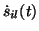and the posterior means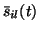and variances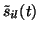of the Gaussians corresponding to different source are most easily solved by making a quadratic approximation for Cf based on the derivatives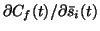and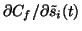, where Cf denotes the sum of terms of the form (27).

The usefulness of this approximation is based on the fact that the cost function tries to minimise the misfit between the approximation and the actual posterior. If we can solve the actual posterior and show that it can be described by our parametric approximation, we know that the cost function will be minimised by setting the parameters of the approximation to values corresponding to the actual posterior.

In this case, making a second order approximation for Cf is equivalent to approximating p(X | si(t)) by an unnormalised Gaussian distribution. Since the prior p(si(t)) is a mixture of Gaussians and the posterior will be given by p(si(t) | X) = p(X | si(t)) p(si(t)) / p(X), we notice that the posterior is also a mixture of Gaussians since a Gaussian multiplied with an unnormalised Gaussian will produce another unnormalised Gaussian and the normalising factor p(X) will then make sure that the posterior is a normalised mixture of Gaussians. From the resulting mixture of Gaussians one can then determine the values for,and. In , a similar method without approximations was used for a linear model. Due to the linearity of the mapping,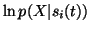is quadratic and no approximations are needed.Next: Avoiding Problems Originating from Up: Update Rules Previous: Update Rules
Harri Lappalainen
2000-03-03